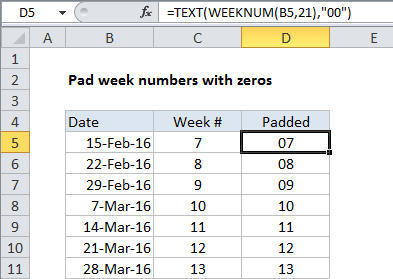## Excel Office

Excel How Tos, Tutorials, Tips & Tricks, Shortcuts

# Pad week numbers with zeros in Excel

To pad week numbers (or any number) with zeros using a formula, you can use the TEXT function.

## Formula

`=TEXT(WEEKNUM(date,type),"00")`## Explanation

In the example show, D5 contains this formula:

`=TEXT(WEEKNUM(B5,21),"00")`

Which returns the string “07”.

Worked Example:   Sum by week number in Excel

### How this formula works

The TEXT function can apply number formats of any kind, including currency, date, percentage, etc. By applying a number format like “00”, “000”, “0000”, you can “pad” numbers with as many zeros as you like. Zeros will only be added where needed.

Worked Example:   Get week number from date in Excel

### Number format only

The TEXT function converts numbers to text as a normal step in applying the number format.

If you are concatenating (joining) the result to another text string, this is fine, but if you just want to apply visual padding to a free-standing number set of numbers, you can apply a custom number format without using a formula.

Worked Example:   TEXT function: Description, Usage, Syntax, Examples and Explanation